Miscellaneous

Chapter 1 Class 12 Relation and Functions
Serial order wise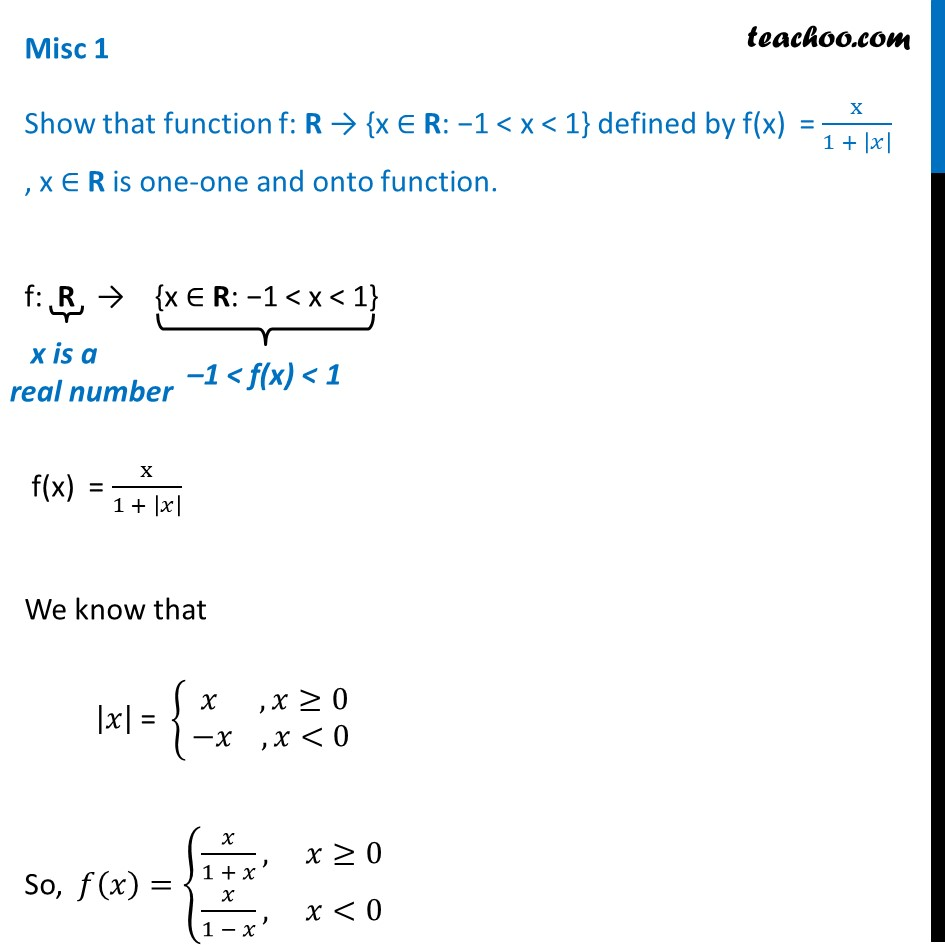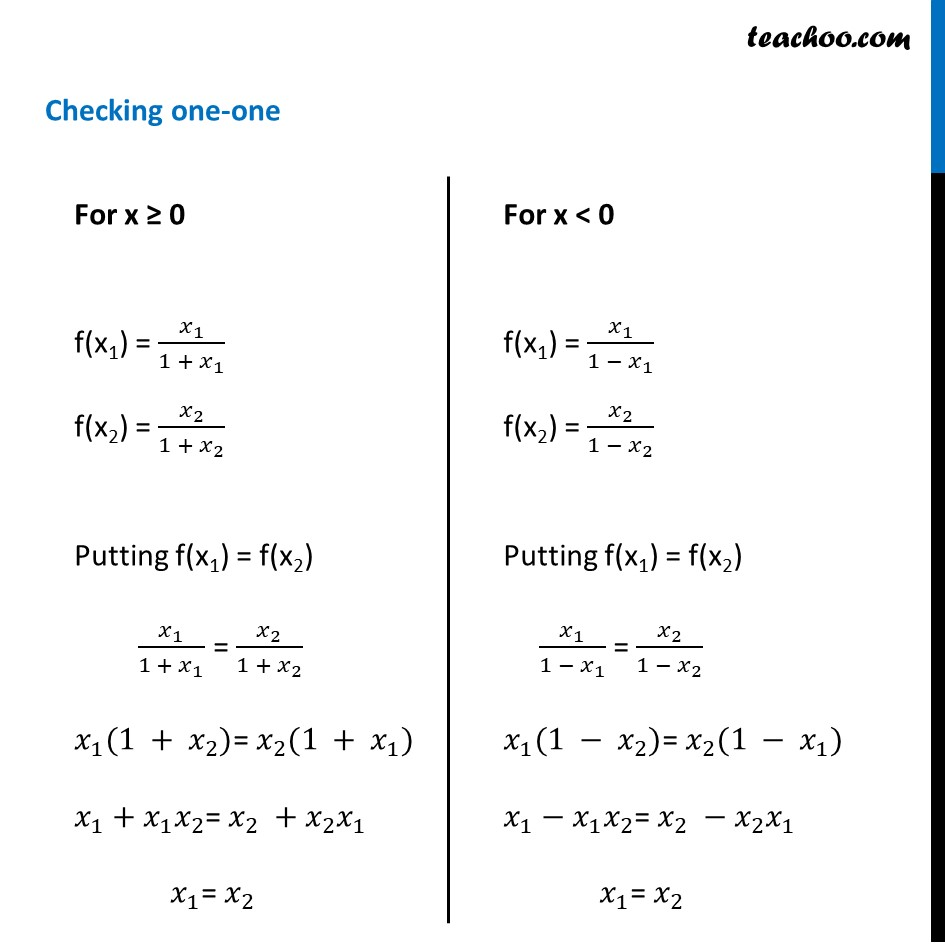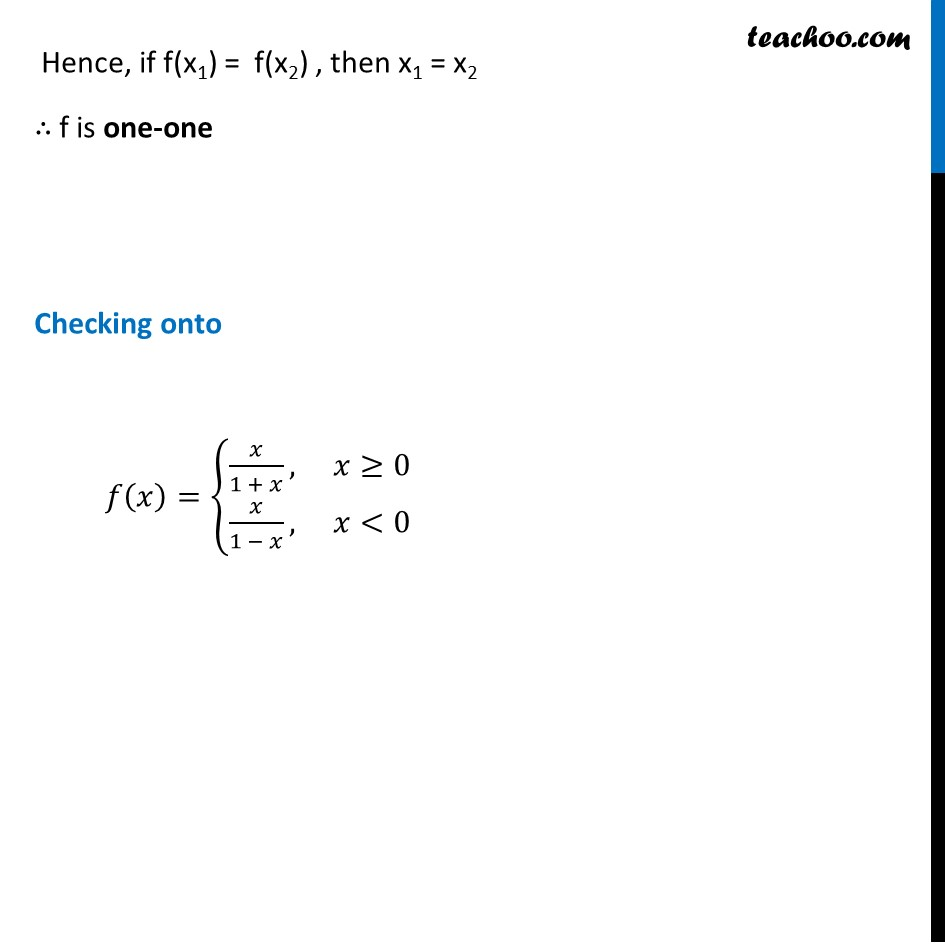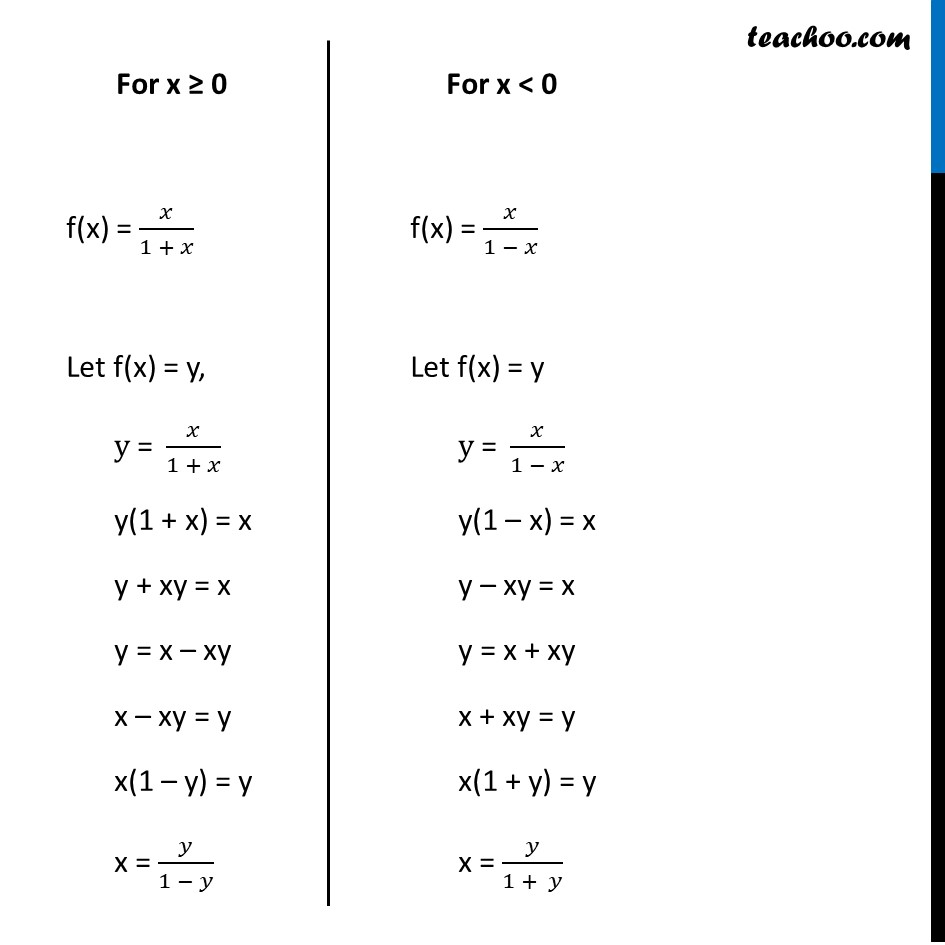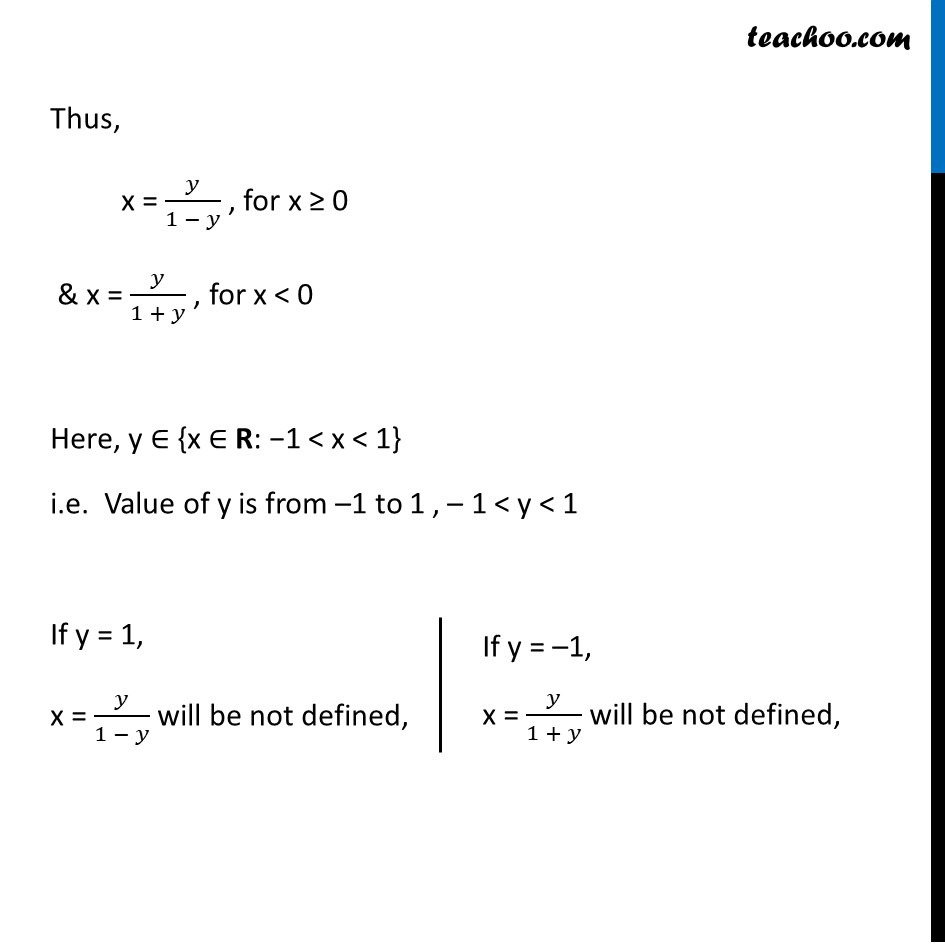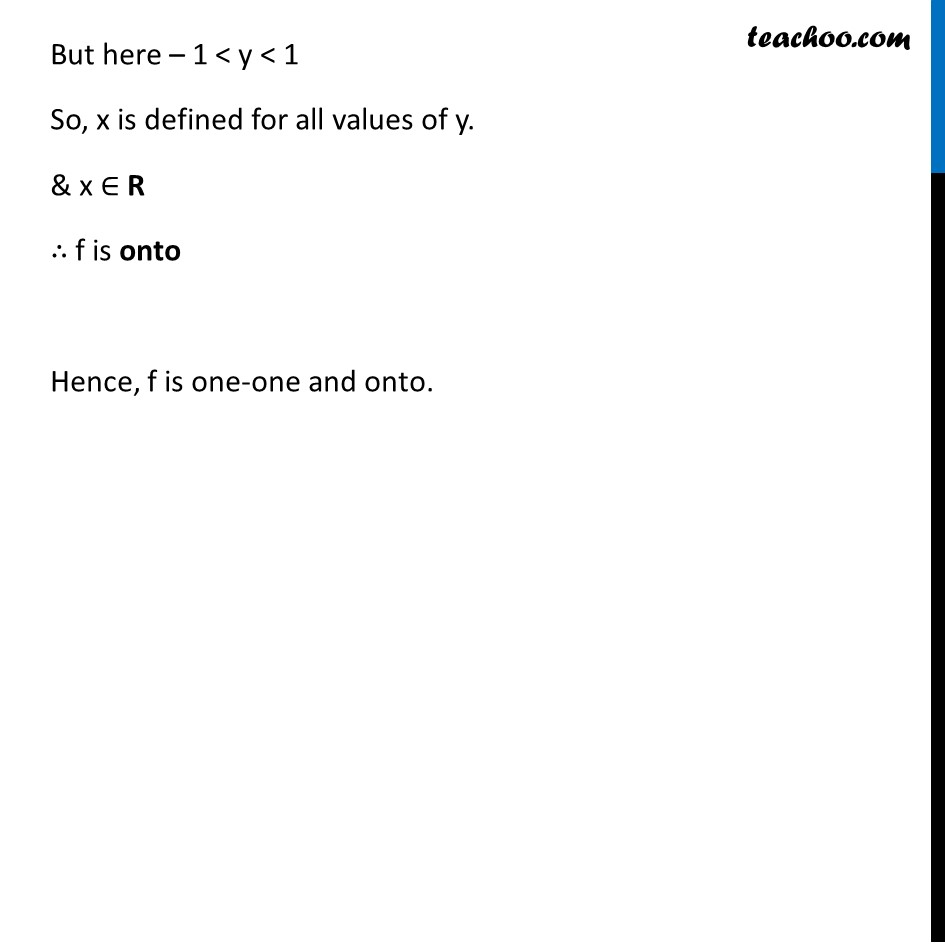Learn in your speed, with individual attention - Teachoo Maths 1-on-1 Class

### Transcript

Misc 1 Show that function f: R → {x ∈ R: −1 < x < 1} defined by f(x) = x/(1 + |𝑥| ) , x ∈ R is one-one and onto function. f: R → {x ∈ R: −1 < x < 1} f(x) = x/(1 + |𝑥| ) We know that |𝑥| = {█( 𝑥 , 𝑥≥0 @−𝑥 , 𝑥<0)┤ So, 𝑓(𝑥)={█(𝑥/(1 + 𝑥), 𝑥≥0@&𝑥/(1 − 𝑥), 𝑥<0)┤ For x ≥ 0 f(x1) = 𝑥_1/(1 + 𝑥_1 ) f(x2) = 𝑥_2/(1 + 𝑥_2 ) Putting f(x1) = f(x2) 𝑥_1/(1 + 𝑥_1 ) = 𝑥_2/(1 + 𝑥_2 ) 𝑥_1 (1 + 𝑥_2)= 𝑥_2 (1 + 𝑥_1) 𝑥_1+𝑥_1 𝑥_2= 𝑥_2 +𝑥_2 𝑥_1 𝑥_1= 𝑥_2 For x < 0 f(x1) = 𝑥_1/(1 − 𝑥_1 ) f(x2) = 𝑥_2/(1 − 𝑥_2 ) Putting f(x1) = f(x2) 𝑥_1/(1 − 𝑥_1 ) = 𝑥_2/(1 − 𝑥_2 ) 𝑥_1 (1 − 𝑥_2)= 𝑥_2 (1 − 𝑥_1) 𝑥_1−𝑥_1 𝑥_2= 𝑥_2 −𝑥_2 𝑥_1 𝑥_1= 𝑥_2 Hence, if f(x1) = f(x2) , then x1 = x2 ∴ f is one-one Checking onto 𝑓(𝑥)={█(𝑥/(1 + 𝑥), 𝑥≥0@&𝑥/(1 − 𝑥), 𝑥<0)┤ For x ≥ 0 f(x) = 𝑥/(1 + 𝑥) Let f(x) = y, "y = " 𝑥/(1 + 𝑥) y(1 + x) = x y + xy = x y = x – xy x – xy = y x(1 – y) = y x = 𝑦/(1 − 𝑦) For x < 0 f(x) = 𝑥/(1 − 𝑥) Let f(x) = y "y = " 𝑥/(1 − 𝑥) y(1 – x) = x y – xy = x y = x + xy x + xy = y x(1 + y) = y x = 𝑦/(1 + 𝑦) Thus, x = 𝑦/(1 − 𝑦) , for x ≥ 0 & x = 𝑦/(1 + 𝑦) , for x < 0 Here, y ∈ {x ∈ R: −1 < x < 1} i.e. Value of y is from –1 to 1 , – 1 < y < 1 If y = 1, x = 𝑦/(1 − 𝑦) will be not defined, If y = –1, x = 𝑦/(1 + 𝑦) will be not defined, But here – 1 < y < 1 So, x is defined for all values of y. & x ∈ R ∴ f is onto Hence, f is one-one and onto.